Surabhi S — Updated On September 22nd, 2022

This article was published as a part of the Data Science Blogathon

## Introduction

Machine Learning is a field of technology developing with immense abilities and applications in automating tasks, where neither human intervention is needed nor explicit programming.

The power of ML is such great that we can see its applications trending almost everywhere in our day-to-day lives. ML has solved many problems that existed earlier and have made businesses in the world progress to a great extend.

Today, we’ll go through one such practical problem and build a solution(model) on our own using ML.

Well, we will deploy our built model using Flask and Heroku applications. And in the end, we will have fully working web applications in our hands.

## Why is it important to deploy your model?

Machine Learning models generally aim to be a solution to an existing problem or problems. And at some point in your life, you must have thought that how would your model be a solution and how would people use this? Indeed, people can’t use your notebooks and code directly, and that’s where you need to deploy your model.

You can either deploy your, model, like API or a web service. Here we are using the Flask micro-framework. Flask defines a set of constraints for the web app to send and receive data.

## Care Price Prediction System

We are about to deploy an ML model for car selling price prediction and analysis. This kind of system becomes handy for many people.

Imagine a situation where you have an old car and want to sell it. You may of course approach an agent for this and find the market price, but later may have to pay pocket money for his service in selling your car. But what if you can know your car selling price without the intervention of an agent. Or if you are an agent, definitely this will make your work easier. Yes, this system has already learned about previous selling prices over years of various cars.

So, to be clear, this deployed web application will provide you will the approximate selling price for your car based on the fuel type, years of service, showroom price, the number of previous owners, kilometres driven, if dealer/individual, and finally if the transmission type is manual/automatic. And that’s a brownie point.

Any kind of modifications can also be later inbuilt in this application. It is only possible to later make a facility to find out buyers. This a good idea for a great project you can try out. You can deploy this as an app like OLA or any e-commerce app. The applications of Machine Learning don’t end here. Similarly, there are infinite possibilities that you can explore. But for the time being, let me help you with building the model for Car Price Prediction and its deployment process.

### Importing Dataset

Dataset is attached in the GitHub folder. Check here

The data consist of 300 rows and 9 columns. Since our target is to find the selling price, the target attribute y is also selling price, remaining features are taken for analysis and predictions.

Python Code:

### Feature Engineering

The data. corr() will give you an intuition on the correlation between all attributes in the dataset. More correlated features can be removed since they can lead to overfitting of the model.

```data = data.drop(['Car_Name'], axis=1)
data['current_year'] = 2020 data['no_year'] = data['current_year'] - data['Year']
data = data.drop(['Year','current_year'],axis = 1)
data = pd.get_dummies(data,drop_first=True)
data = data[['Selling_Price','Present_Price','Kms_Driven','no_year','Owner','Fuel_Type_Diesel','Fuel_Type_Petrol',
'Seller_Type_Individual','Transmission_Manual']]
data```

`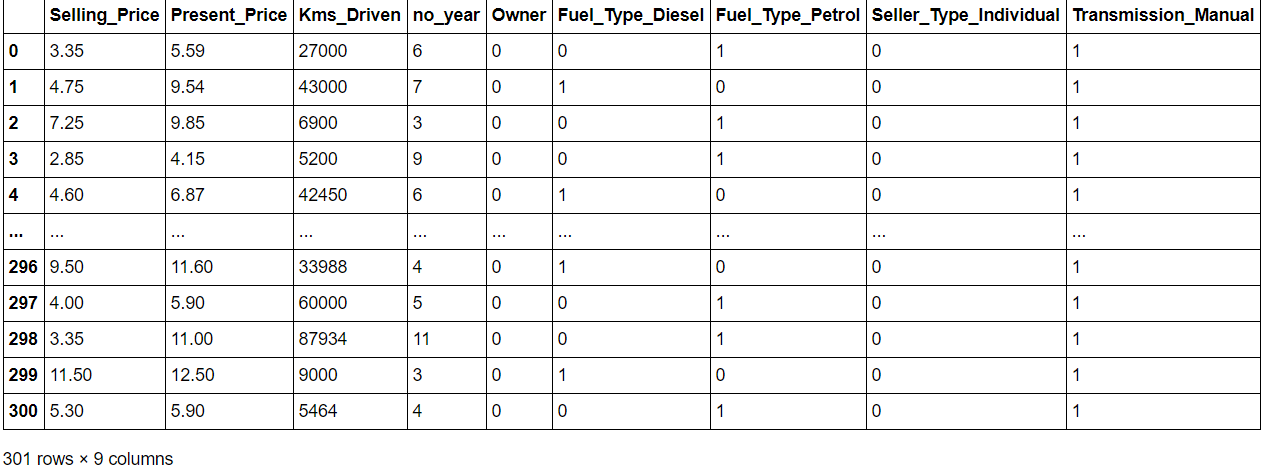`
`data.corr()`
`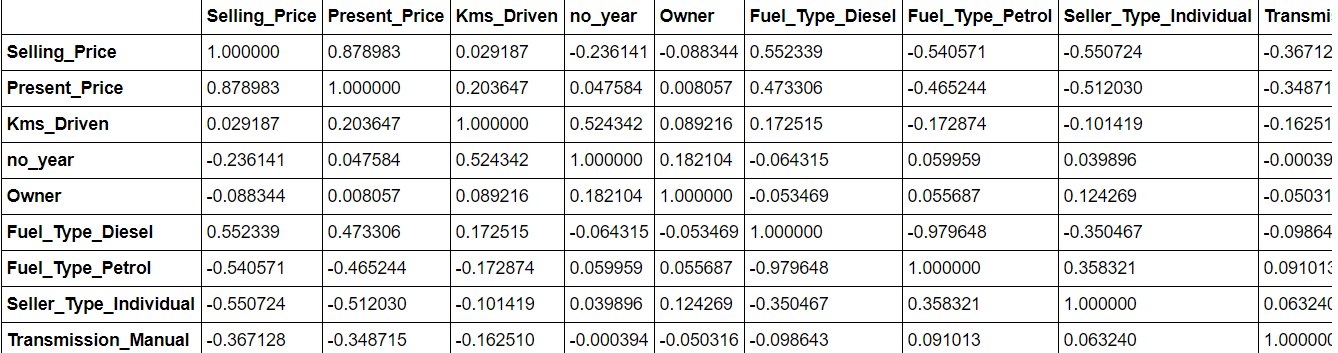`

Next, we are slicing the data into training and test set

```x = data.iloc[:,1:]
y = data.iloc[:,0]```

### Finding out feature importance to eliminate unwanted features

The extratressregressor library allows you to view feature importances and thereby remove the less important features from the data. It is always advised to remove the unnecessary feature because they can definitely yield better accuracy scores.

```from sklearn.ensemble import ExtreesRegressor
model = ExtraTreesRegressor()
model.fit(x,y)```
`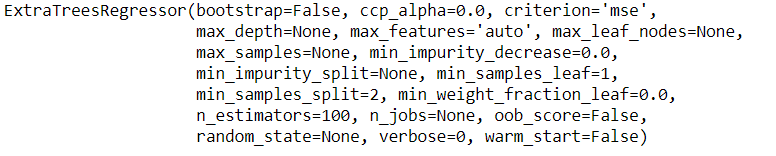`
`model.feature_importances_`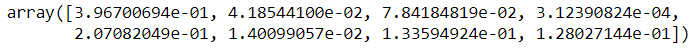### Hyperparameter Optimization

This is done so as to get the optimal values for use in our model, this can also to an extend

help to get good results in prediction

```n_estimators = [int(x) for x in np.linspace(start = 100, stop = 1200,num = 12)]
max_features = ['auto','sqrt']
max_depth = [int(x) for x in np.linspace(5,30,num = 6)]
min_samples_split = [2,5,10,15,100]
min_samples_leaf  = [1,2,5,10]```
```grid = {'n_estimators': n_estimators,
'max_features': max_features,
'max_depth': max_depth,
'min_samples_split': min_samples_split,
'min_samples_leaf': min_samples_leaf}
print(grid)```

# Output

```{'n_estimators': [100, 200, 300, 400, 500, 600, 700, 800, 900, 1000, 1100, 1200],
'max_features': ['auto', 'sqrt'],
'max_depth': [5, 10, 15, 20, 25, 30],
'min_samples_split': [2, 5, 10, 15, 100],
'min_samples_leaf': [1, 2, 5, 10]} ```

### Train Test Split

```from sklearn.model_selection import train_test_split    #importing train test split module
x_train, x_test,y_train,y_test = train_test_split(x,y,random_state=0,test_size=0.2)```

### Training the model

We have used the random forest regressor to predict the selling prices since this is a regression problem and that random forest uses multiple decision trees and has shown good results for my model.

```from sklearn.ensemble import RandomForestRegressor model = RandomForestRegressor()
hyp = RandomizedSearchCV(estimator = model,
param_distributions=grid,
n_iter=10,
scoring= 'neg_mean_squared_error'
cv=5,verbose = 2,
random_state = 42,n_jobs = 1)
hyp.fit(x_train,y_train)```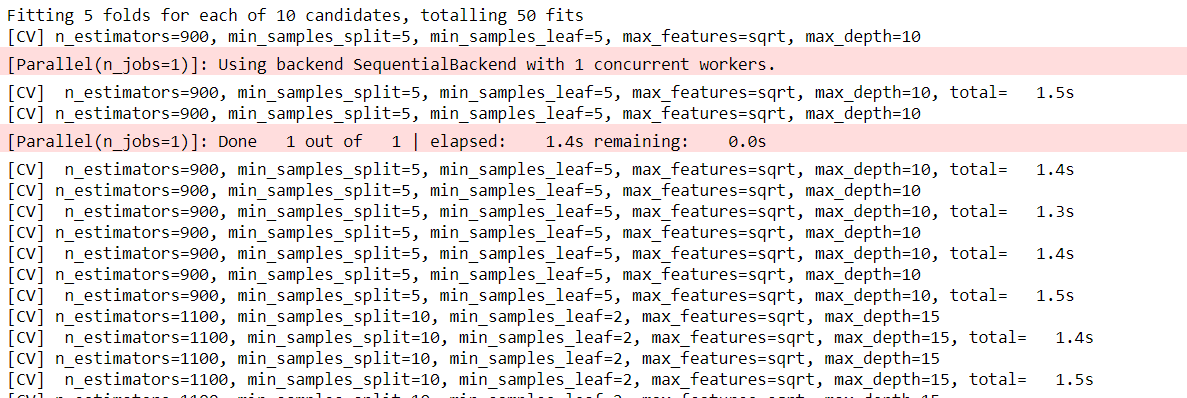hyp is a model created using the optimal hyperparameters obtained through randomized search cross-validation

### Output

Now we finally use the model to predict the test dataset.

```y_pred = hyp.predict(x_test)
y_pred```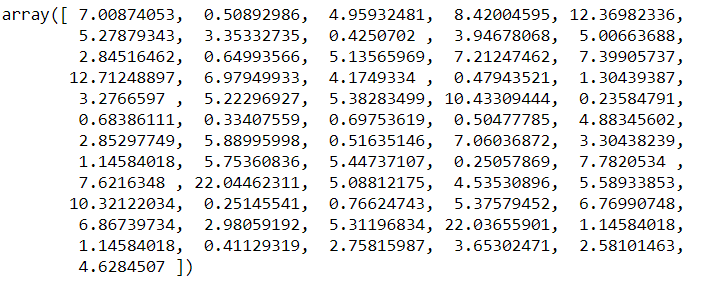To use the Flask framework for deployment, it is necessary to pack this whole model and import it into the python file for creating web applications. Hence we dump our model into the pickle file using the given code.

```import pickle
file = open("file.pkl", "wb") # opening a new file in write mode
pickle.dump(hyp, file) # dumping created model into a pickle file```

Full Code

What we need is a web application containing a form to take the input from the user, and return the predictions from the model. So we’ll develop a simple web app for this. The front end is made using simple HTML and CSS. I advise you to go through the basics of web development to understand the meaning of code written for the front end. It would be also great if you have an understanding of the flask framework. Go through this video if you are new to FLASK.

Let me explain to you, in brief, what I have coded using FLASK.

So let’s start the code by importing all the required libraries used here.

```from flask import Flask, render_template, request
import pickle
import requests
import numpy as np```
As you know we have to import the saved model here in order to do the predictions of the data’s provided by the user. So we’re importing the saved model
`model = pickle.load(open("model.pkl", "rb"))`
Now let’s go into the code for creating the actual flask app.
```app = Flask(_name_)
@app.route("/") # this will direct  us to the home page when we click our web app link
def home():
@app.route("/predict", methods = ["POST"]) # this works when the user click the prediction button
def predict():
year = int(request.form["year"]) # taking year input from the user
tot_year = 2020 - year
present_price = float(request.form["present_price"]) #taking the present prize
fuel_type = request.form["fuel_type"] # type of fuel of car
# if loop for assigning numerical values
if fuel_type == "Petrol":
fuel_P = 1
fuel_D = 0
else:
fuel_P = 0
fuel_D = 1
kms_driven = int(request.form["kms_driven"]) # total driven kilometers of the car
transmission = request.form["transmission"] # transmission type
# assigning numerical values
if transmisson == "Manuel":
transmission_manual = 1
else:
transmission_manual = 0
seller_type = request.form["seller_type"] # seller type
if seller_type == "Individual":
seller_individual = 1
else:
seller_individual = 0
owner = int(request.form["owner"])  # number of owners
values = [[
present_price,
kms_driven,
owner,
tot_year,
fuel_D,
fuel_P,
seller_individual,
transmission_manual
]]
# created a list of all the user inputed values, then using it for prediction
prediction = model.predict(values)
prediction = round(prediction,2)
# returning the predicted value inorder to display in the front end web application
return render_template("home.html", pred = "Car price is {} Lakh".format(float(prediction)))
if _name_ == "_main_":
app.run(debug = True)```

## Deploying Using Heroku

All you need to do is connect your GitHub repository containing all necessary files for the project with Heroku. For all those who don’t know what Heroku is, Heroku is a platform that enables developers to build, run, and operate applications in the cloud.

This is the link to the web app that I have created using the Heroku platform. So, we have seen the building and deployment process of a machine learning model. You can also do it, learn more and never hesitate to try out new things and develop.

https://car-price-analysis-app.herokuapp.com/

##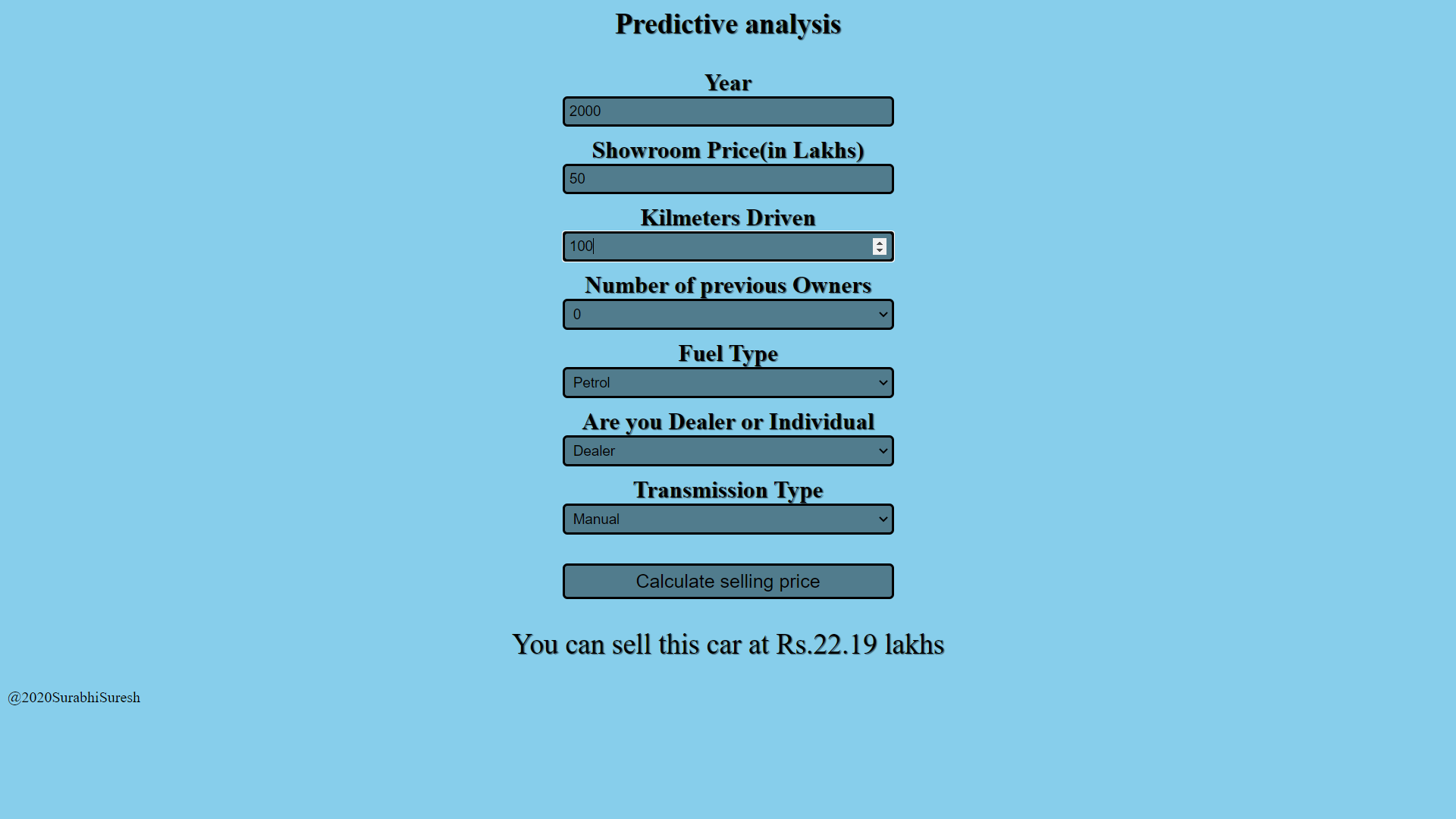Conclusion

So, we have seen the building and deployment process of a machine learning model. You can also do it, learn more and never hesitate to try out new things and develop. Feel free to connect with me on linked in.

Thank you

The media shown in this article are not owned by Analytics Vidhya and is used at the Author’s discretion.